## Sunday, March 28, 2010

### Irodov Problem 3.20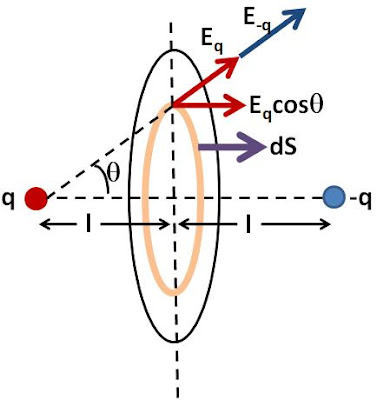Consider an infinitesimally small ring section of radius r and width dr in the central plane between the negative and positive charges. The area vector of this infinitesimally thin ring section is given by,The magnitude electric field at all points over this ring due to the positive charge will be,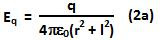The electric field due to the negative charge will be the same as that of the positive charge,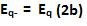The component of electric field along the x-axis (direction of the ring section area vector) if given by,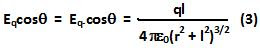Hence, the total flux can be obtained by intergrating the horizontal component of the electric field over the entire disc as,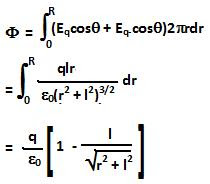1.This comment has been removed by the author.

2.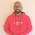3.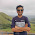4.The net electric field at any point on disc is KP/r^3 where p is dipole moment and is perpendicular to disc.

5.The net electric field at any point on disc is KP/r^3 where p is dipole moment and is perpendicular to disc.

6.Your aumption is wrong

7.This comment has been removed by the author.

8.Very understandable good job